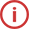#### FilterMinimum order amount: 500 UAH
Quantity of SMD: multiples 100 pcs

An analog-to-digital converter is an analog-to-digital converter, or ADC for short. It is a device that converts a continuous analog signal to a discrete digital signal.

### Sound (voice) and its transformation.

An analog signal is continuous in the time domain, so it can be converted to a series of continuous digital signals. This requires a parameter definition to indicate the rate of the new digital signal sampled from the analog signal. This frequency is called the sampling rate or the sampling rate of the converter.

It can collect continuously changing signals with limited bandwidth (that is, measure and store the signal value every time), and then restore the converted discrete signal to the original signal through interpolation. The accuracy of this process is limited by the quantization error. However, only when the sampling rate is more than twice the signal frequency can the original signal be accurately reconstructed. This law is reflected in the discretization theorem.

Since the actual A / D converter cannot perform full conversion in real time, some additional techniques need to be used to keep it constant while converting the input signal. A sample and hold scheme is commonly used. In most cases, a capacitor can be used to store the analog input voltage, and a switch or gate circuit can be used to turn the capacitor and the input signal on and off. Many ADCs already include such ​​a sampling and storage subsystem.